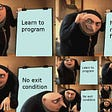# Union Find and Prime Factorization

• If two numbers have common factor, union them
• During the union process, maintain a set size as the final answer is asking for the set size.

# Plain solution

`class UF{    public:    vector<int> roots;    vector<int> sizes;    UF(int n){        roots.assign(n, 0); for (int i = 0; i < n; ++i)roots[i] = i;        sizes.assign(n, 1);}    int find_set(int x){        return x == roots[x]? x: roots[x] = find_set(roots[x]);    }    void union_set(int x, int y){        int root_x = find_set(x), root_y = find_set(y);        if (root_x == root_y)return;        sizes[root_x] += sizes[root_y];        roots[root_y] = root_x;    }    int get_set_size(int x){        return sizes[x];    }};class Solution {    public:    int largestComponentSize(vector<int>& nums) {        const int n = nums.size();        UF uf(n);        unordered_map<int, vector<int>> graph;        for (int i = 0; i < n; ++i){            int num = nums[i];            for (int factor = 1; factor <= int(sqrt(num)); ++factor){                if (num % factor == 0){                    if(factor != 1)graph[factor].push_back(i);                    if(num / factor != 1)graph[num / factor].push_back(i);                }            }        }        for (auto [k, v]: graph){            const int m = v.size();            for (int i = 1; i < m ; ++i){                uf.union_set(v, v[i]);            }        }        int ret = 0;        for (int i = 0; i < n; ++i){            ret = max(ret, uf.get_set_size(i));        }        return ret;    }};`

# Speed up by using Prime Factorization

`class UF{    public:    vector<int> roots;    vector<int> sizes;    UF(int n){        roots.assign(n, 0); for (int i = 0; i < n; ++i)roots[i] = i;        sizes.assign(n, 1);            }    int find_set(int x){        return x == roots[x]? x: roots[x] = find_set(roots[x]);    }    void union_set(int x, int y){        int root_x = find_set(x), root_y = find_set(y);        if (root_x == root_y)return;        sizes[root_x] += sizes[root_y];        roots[root_y] = root_x;    }    int get_set_size(int x){        return sizes[x];    }};vector<int> primeFactors(int n){        vector<int>ret;    // Print the number of 2s that divide n    while (n % 2 == 0)    {        ret.push_back(2);        n = n/2;    }     // n must be odd at this point. So we can skip    // one element (Note i = i +2)    for (int i = 3; i <= sqrt(n); i = i + 2)    {        // While i divides n, print i and divide n        while (n % i == 0)        {            ret.push_back(i);            n = n/i;        }    }     // This condition is to handle the case when n    // is a prime number greater than 2    if (n > 2)        ret.push_back(n);    return ret;}class Solution {public:    int largestComponentSize(vector<int>& nums) {        const int n = nums.size();        UF uf(n);        unordered_map<int, vector<int>> graph;        for (int i = 0; i < n; ++i){            int num = nums[i];            auto factors = primeFactors(num);            for (auto &factor: factors)graph[factor].push_back(i);        }        for (auto [k, v]: graph){            const int m = v.size();            for (int i = 1; i < m ; ++i){                    uf.union_set(v, v[i]);            }        }        int ret = 0;        for (int i = 0; i < n; ++i){            ret = max(ret, uf.get_set_size(i));        }        return ret;    }};`

# Run time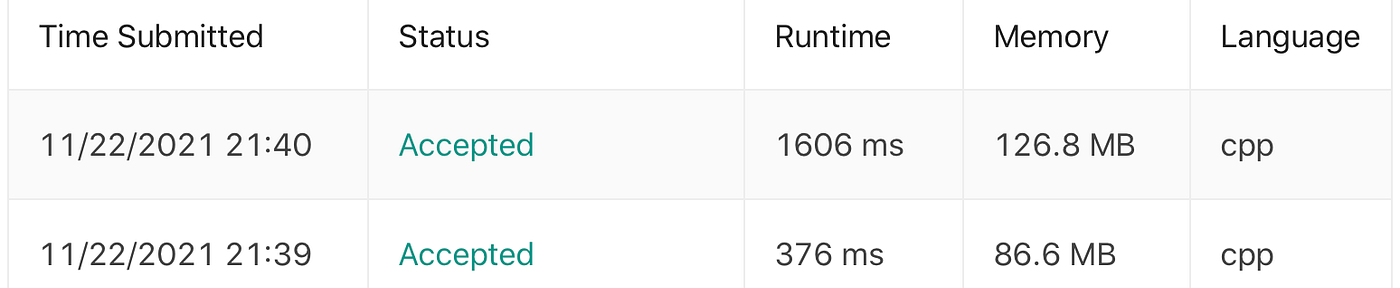Time difference Top submission is the plain version and the bottom one is using the Prime Factorization version

# Reference

--

--

--

## More from Jimmy Shen

Data Scientist/MLE/SWE @takemobi

Love podcasts or audiobooks? Learn on the go with our new app.

## Eth 2.0 Dev Update #49 — “Multiclient Testnet + Security Audit”## Imposter Syndrome Kept Me out of Tech for 16 Years## Overview of Apache Spark (Data Engineer starter pack — Part 3)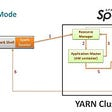## A Rock-Paper-Scissors terminal game in Python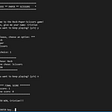## Vanilla Mob Variants | Minecraft PE Texture — MCPE AddOns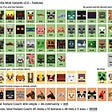## CI/CD pipelines in low-code / no-code environments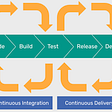## Build log: Making a frame-less news desktop app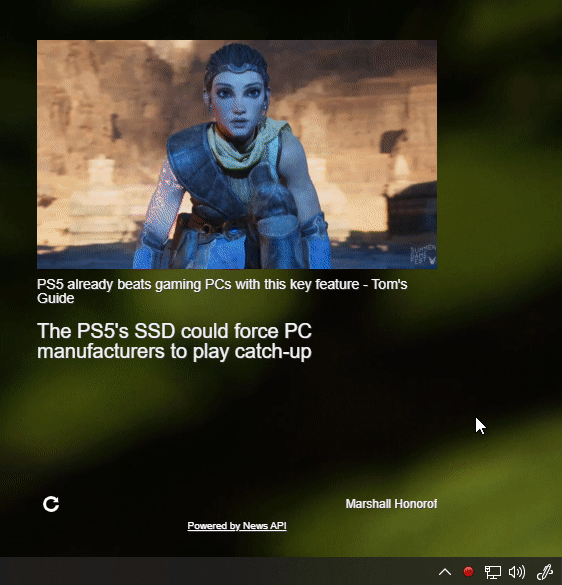## How To Publish App On Play Store Free With Academy For App Success## Jimmy Shen

Data Scientist/MLE/SWE @takemobi

## How to rotate image (matrix) to 90° in-place using O(n) time## A series on Recursion